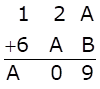Solve Cryptarithms:Asked by Sakshi | 1 year ago |  87

##### Solution :-

Firstly let us solve for unit’s place,

A + B = 9  (1)

With this condition we know that sum of 2 digits can be greater than 18.

So, there is no need to carry one from ten’s place.

Now let us solve for ten’s place,

2 + A = 0

Which means A = -2 which is never possible

Hence, 2 + A > 9

Now, there should be one carry in hundred’s place and hence we need to subtract 10 from ten’s place,

i.e., 2 + A – 10 = 0

A = 10-2 = 8

Now, substituting A=8 in 1,

A + B = 9

8 + B = 9

B = 9 – 8

B = 1

A = 8 and B = 1

Answered by Aaryan | 1 year ago

### Related Questions

#### Which of the following numbers are divisible by 11

Which of the following numbers are divisible by 11:

(i) 10835

(ii) 380237

(iii) 504670

(iv) 28248

#### In each of the following replace * by a digit so that the number formed is divisible by 11:

In each of the following replace * by a digit so that the number formed is divisible by 11:

(i) 64 × 2456

(ii) 86 × 6194

#### In each of the following replace * by a digit so that the number formed is divisible by 6:

In each of the following replace * by a digit so that the number formed is divisible by 6:

(i) 97 × 542

(ii) 709 × 94

#### In each of the following replace × by a digit so that the number formed is divisible by 9:

In each of the following replace × by a digit so that the number formed is divisible by 9

(i) 49 × 2207

(ii) 5938 × 623